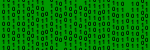#### You may also like### 8 Methods for Three by One

This problem in geometry has been solved in no less than EIGHT ways by a pair of students. How would you solve it? How many of their solutions can you follow? How are they the same or different? Which do you like best?### Rots and Refs

Follow hints using a little coordinate geometry, plane geometry and trig to see how matrices are used to work on transformations of the plane.### Reflect Again

Follow hints to investigate the matrix which gives a reflection of the plane in the line y=tanx. Show that the combination of two reflections in intersecting lines is a rotation.

# The Matrix

##### Age 16 to 18Challenge LevelOne of the ways to work with transformations is to use a matrix. If you have not met matrices before don't be put off, they are very easy. In this question you will use some simple matrices for rotations and reflections and see how they work.

First you need to know how to multiply a matrix like

$\left( \begin{array}{cc} a & b \\ c & d \end{array} \right)$ by the vector $\left( \begin{array}{c} x \\ y \end{array} \right)$

to give the image of the point $(x,y)$. This multiplication is defined as follows: $$\left( \begin{array}{cc} a & b \\ c & d \end{array} \right) \left( \begin{array}{c} x \\ y \end{array} \right)$$ $$\left( \begin{array}{cc} a & b \\ c & d \end{array} \right) \left( \begin{array}{c} x \\ y \end{array} \right) = \left( \begin{array}{c} ax+by \\ cx+dy \end{array} \right)$$ Find the images of the points $(1,0)$ and $(0,1)$ under the transformation given by the matrix $$\left( \begin{array}{cc} a & b \\ c & d \end{array} \right)$$

Describe the effect on the plane of the four transformations where $b=c=0$ and $a$ and $d$ take all possible combinations of the values $\pm 1$. Now describe the effect on the plane of the four transformations where $a=d=0$ and $b$ and $c$ take all possible combinations of the values $\pm 1$

Explain why transformations have the same effect on the whole plane as on the unit square with vertices $(0,0),\ (0,1),\ (1,1),\ (1,0)$.